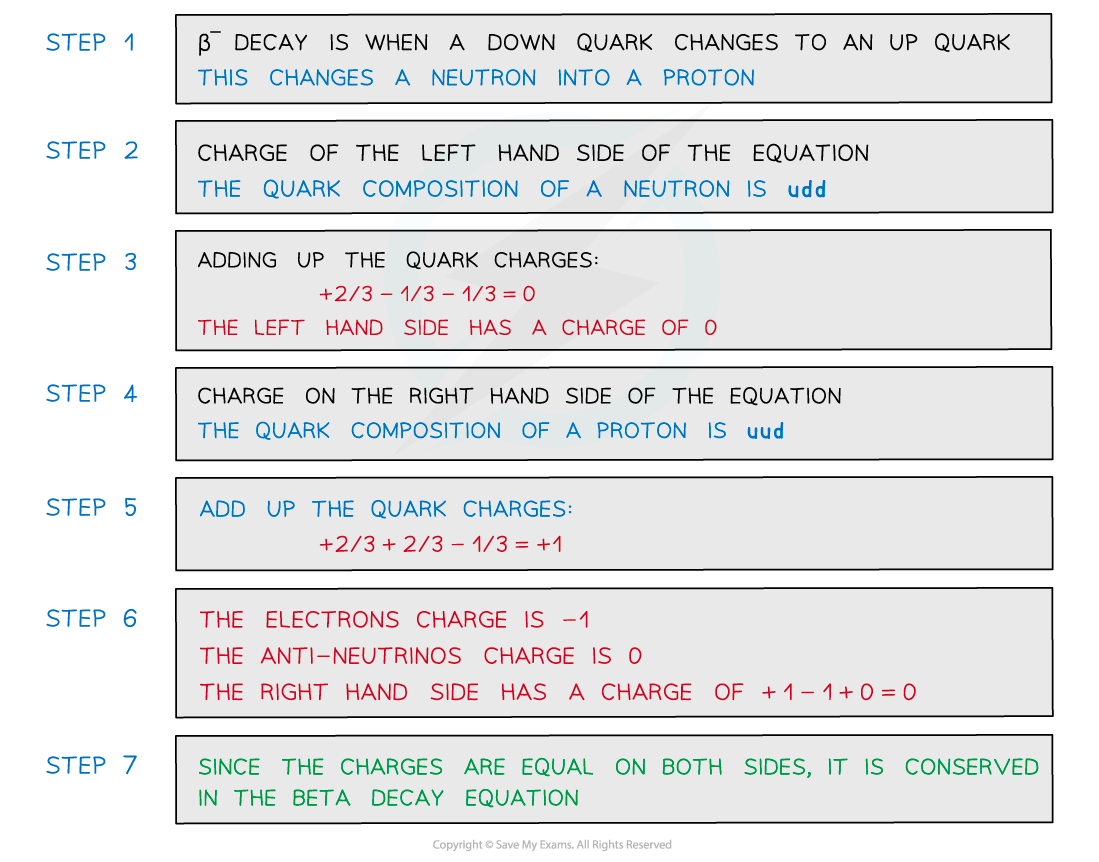# IB DP Physics: HL复习笔记7.3.2 Quarks & Leptons

### Quarks & Leptons

#### Quarks

• Quarks are fundamental particles that make up other subatomic particles such as protons and neutrons
• Protons and neutrons are in a category of particles called hadrons

• Fundamental means that quarks are not made up of any other particles. Another example is electrons
• Quarks have never been observed on their own, they’re either in pairs or groups of three
• There are six flavours (types) of quarks that exist: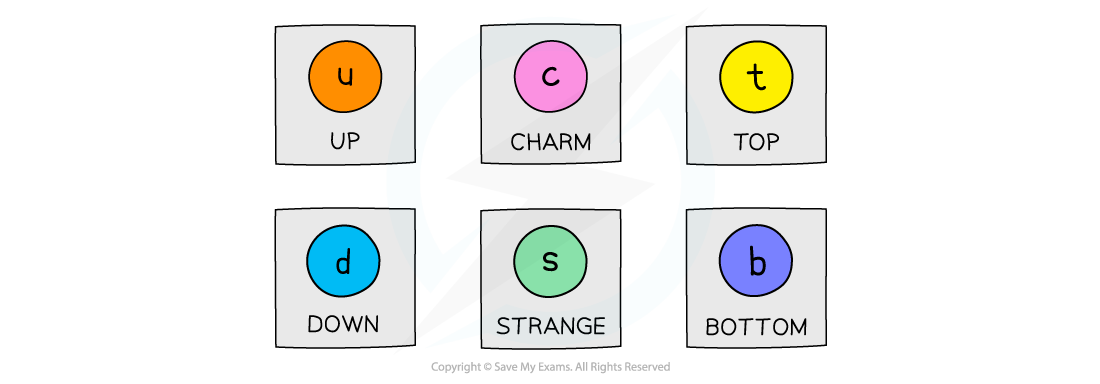The six flavours of quarks

• The charge of a hadron is determined by the sum of the charges of its quarks
• Each flavour of quark has a certain relative charge: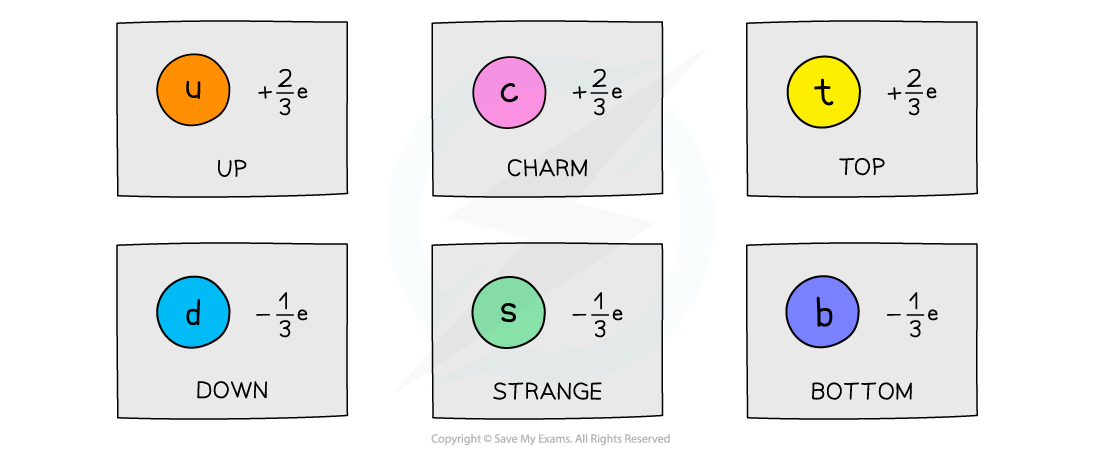Each flavour of quark has a charge of either +⅔e or -⅓e

• For example, a proton is made up of two up quarks and a down quark. Adding up their charges gives the charge of a proton:

+⅔e + ⅔e - ⅓e = +1e

• The equivalent antiparticle of the quark is the anti-quark
• These are identical to quarks except with opposite relative charges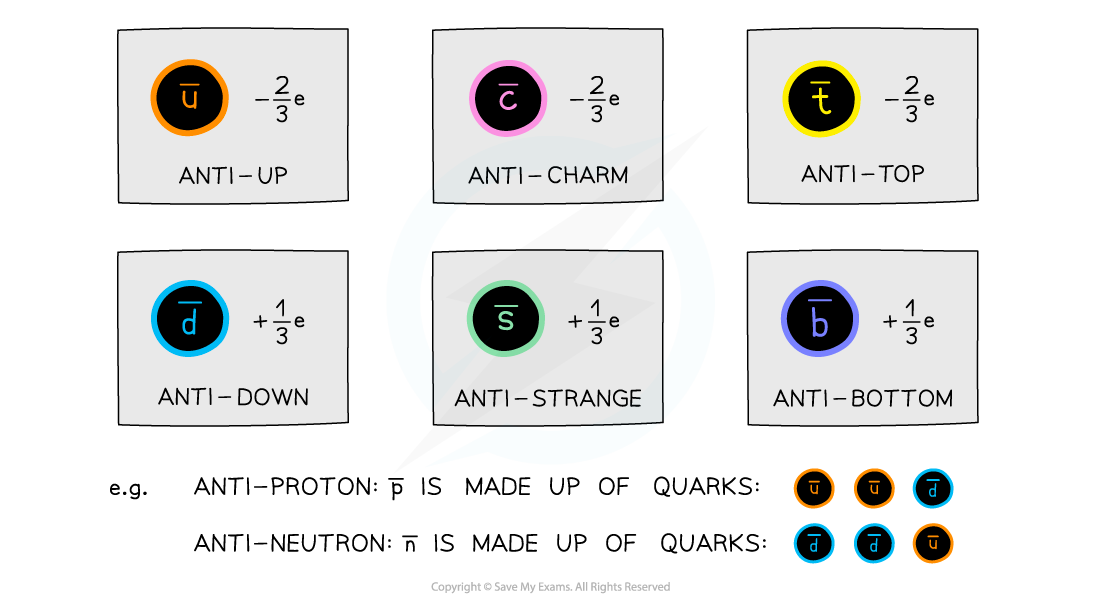Each flavour of anti-quark has a charge of either -⅔e or +⅓e. The quark composition of anti-protons and anti-neutrons changes to anti-quarks

• Quarks have a baryon number of +1/3
• Anti-quarks having a baryon number of –1/3
• Strange quarks have a strangeness of –1
• Anti-strange quarks have a strangeness of +1
• This is unique to the strange quark

#### Worked Example

Particles are made up of a combination of three quarks or two quarks. Which quark combination would not give a particle a charge of -1 or 0?

A. up, strange, strange

B. charm, charm, down

C. top, anti-up

D. anti-up, anti-up, anti-strange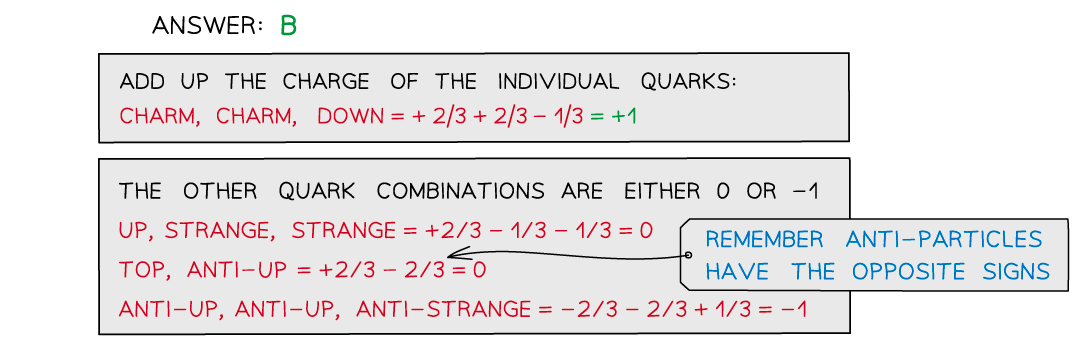#### Worked Example

A K- particle has a strangeness of –1. Determine the quark structure of this particle.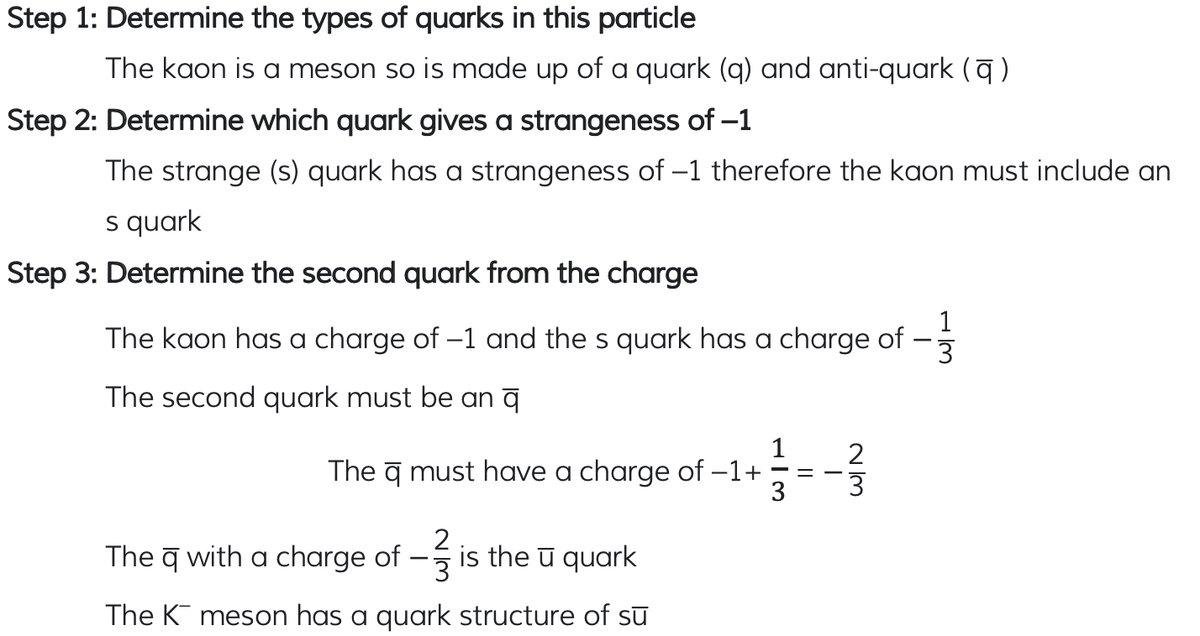#### Leptons

• Leptons are a group of fundamental (elementary) particles
• This means they are not made up of any other particles (no quarks)
• There are six leptons altogether: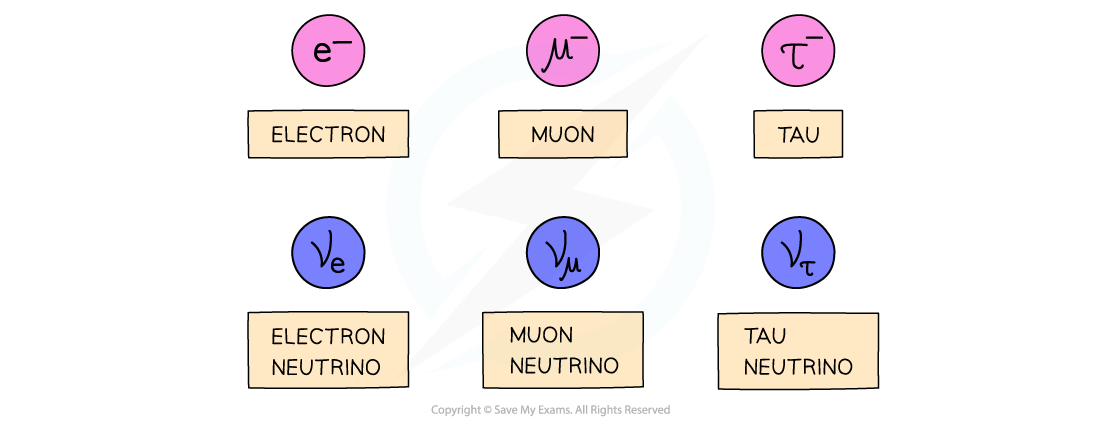The six leptons are all fundamental particles

• The muon and tau particle are very similar to the electron but with slightly larger mass
• Electrons, muon, and tau particles all have a charge of -1e and a mass of 0.0005u
• There are three flavours (types) of neutrinos (electron, muon, tau)
• Neutrinos are the most abundant leptons in the universe
• They have no charge and negligible mass (almost 0)
• Leptons interact with the weak interaction, electromagnetic and gravitational forces
• However, they do not interact with the strong force
• Although quarks are fundamental particles too, they are not classed as leptons
• Leptons do not interact with the strong force, whilst quarks do

#### Worked Example

Circle all the anti-leptons in the following decay equation.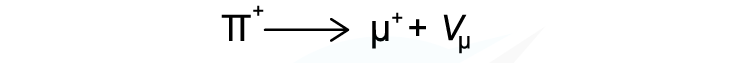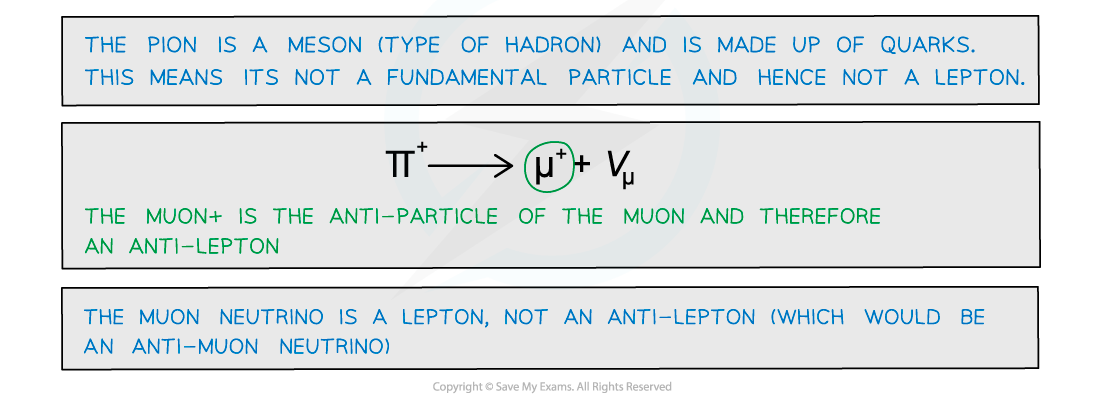#### Lepton Number

• Similar to baryon number, the lepton number, L is the number of leptons in an interaction
• L depends on whether the particle is a lepton, anti-lepton or neither
• Leptons have a lepton number L = +1
• Anti-leptons have a lepton number L = –1
• Particles that are not leptons have a lepton number L = 0
• Lepton number is a quantum number and is conserved in all interactions
• This is helpful for knowing whether an interaction is able to happen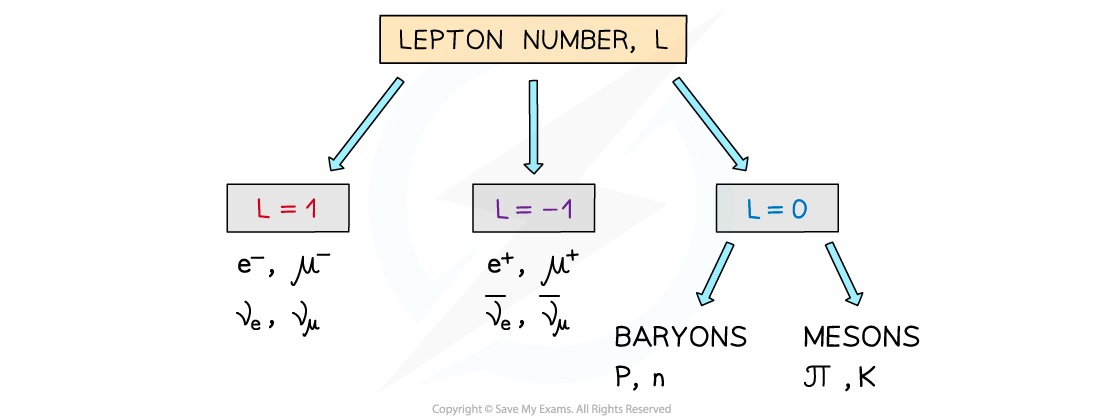The lepton number depends if the particle is a lepton, anti-lepton or neither

#### Worked Example

If the lepton number is conserved in the following decay, identify whether particle X should be a neutrino or anti-neutrinoStep 1: Determine the lepton number of all the particles on both sides of the equation

• 0 + (–1) = 0 + X

Step 2: Identify the lepton number of X

• If the lepton number must be conserved, X must also have a lepton number of –1

Step 3: State the particle X

• Particle X is an anti-neutrino

### Protons & Neutrons

#### Protons and Neutrons

• Protons and neutrons are not fundamental particles. They are each made up of three quarks
• Protons are made up of two up quarks and a down quark
• Neutrons are made up of two down quarks and an up quark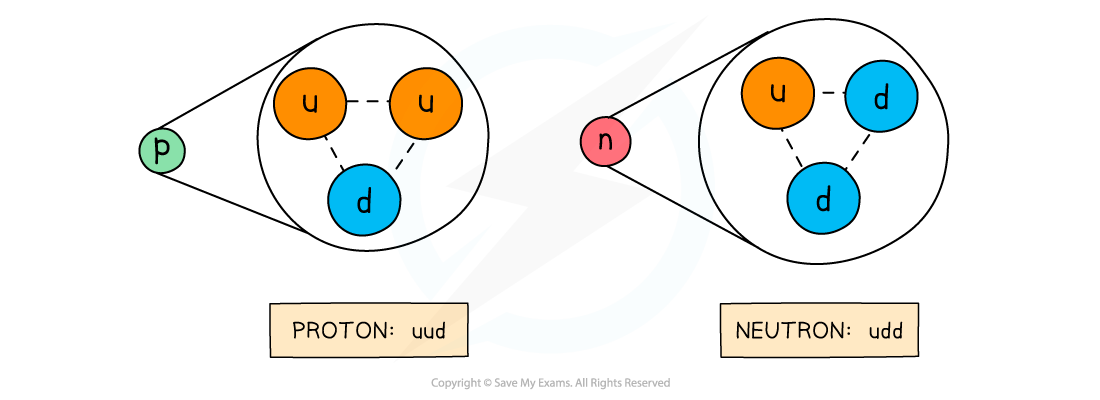Protons and neutrons are made up of three quarks

• You will be expected to remember these quark combinations for exam questions

#### Protons as Baryons

• The proton is the most stable baryon
• This means it has the longest half-life of any baryon and is the particle which other baryons eventually decay to
• It is the most stable baryon because it is also the lightest baryon
• Radioactive decay occurs when heavier particles decay into lighter particles
• A decay of the proton would therefore violate the conservation of baryon number
• It is theorized that the proton has a half-life of around 1032 years and research experiments are still underway that are designed to detect proton decay

#### Worked ExampleStep 1: Calculate number of protons:

• The number of protons is from the proton number = 26 protons

Step 2: Calculate number of neutrons:

• The number of neutrons = nucleon number - proton number = 56 - 26 = 30 neutrons

Step 3: Up quarks in a proton:

• Protons are made up of uud quarks = 2 up quarks

Step 4: Up quarks in a neutron:

• Neutrons are made up of udd quarks = 1 up quark

Step 5: Total number of up quarks:

• 26 protons x 2 up quarks = 52 up quarks
• 30 neutrons x 1 up quark = 30 up quarks
• 52 + 30 = 82 up quarks

#### β– and β+ decay

• Beta decay happens via the weak interaction
• This is one of the four fundamental forces and it’s responsible for radioactive decays

#### Quark Composition: β- decay

• Recall that β- decay is when a neutron turns into a proton emitting an electron and anti-electron neutrino
• More specifically, a neutron turns into a proton because a down quark turns into an up quark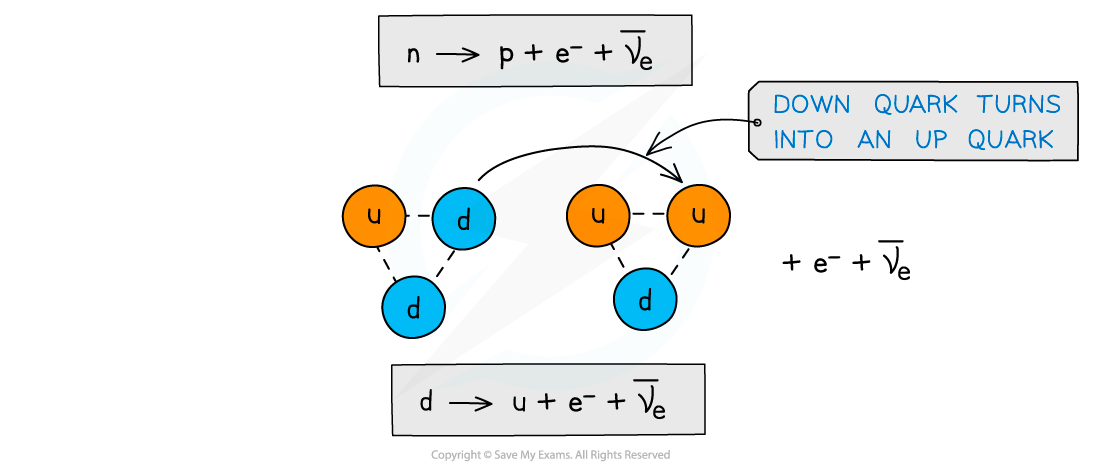Beta minus decay is when a down quark turns into an up quark

#### Quark Composition: β+ decay

• Recall that β+ decay is when a proton turns into a neutron emitting a positron and an electron neutrino
• More specifically, a proton turns into a neutron because an up quark turns into a down quark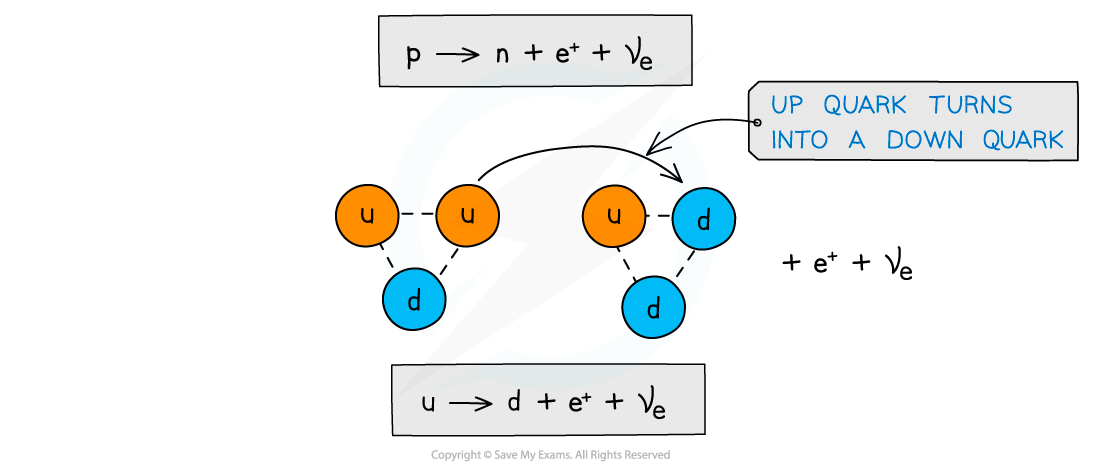Beta plus decay is when an up quark turns into a down quark

#### Worked Example

The equation for β decay is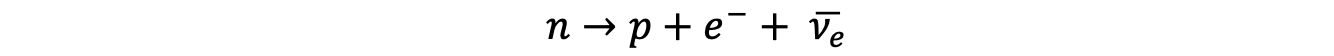Using the quark model of beta decay, prove that the charge is conserved in this equation.